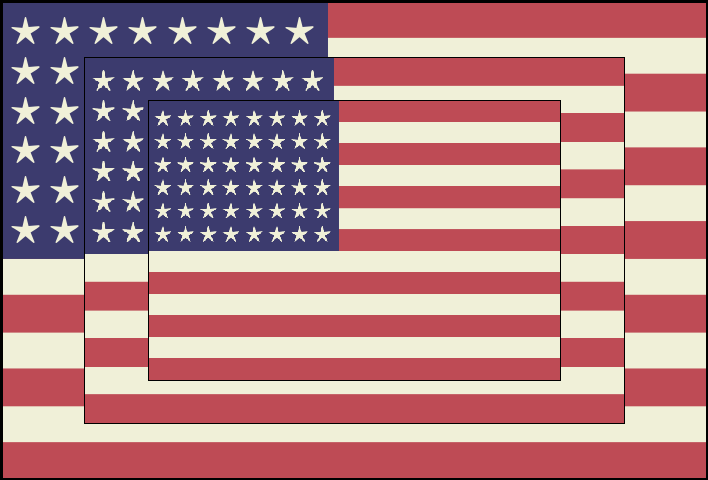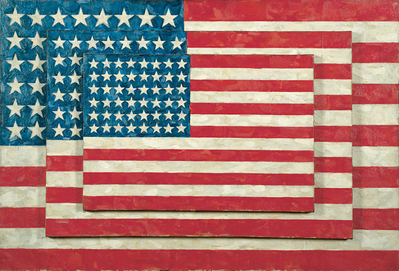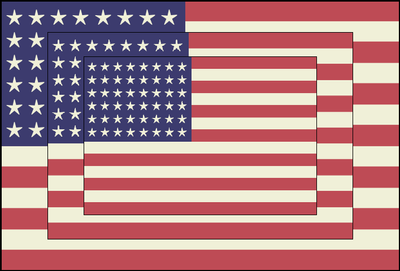## Fun with SAS ODS Graphics: "Three Flags" for July 4thIn advance of Independence Day, here's a quick SAS ODS Graphics bar-and-scatter-plots take on Three Flags by Jasper Johns. Enjoy the holiday, all!

CODE

``````* Fun with SAS ODS Graphics: "Three Flags" for July 4th
A SAS ODS Graphics take on "Three Flags" by Jasper Johns (whitney.org/collection/works/1060);

data stripes; * Generate points for flag stripes;
do y1=1 to 14;
x=mod(y1,2); * Alternate red and white stripes (bar length of 1 or 0);
output;
end;

data canton; * Generate points for canton (blue rectangle in upper left corner);
do y2=1 to 14;
x=.4625*(y2<=7); * Blue over first 7 stripes covering 46.25% of flag width;
output;
end;

data stars; * Generate points for 48 stars (arranged in 6 rows of 8 stars - painted in 1958);
do r=1 to 6;
y3=.79+(r-1)*1.08; * Distribute vertically over first 7 stripes;
do c=1 to 8;
x=.0355+0.96*.4625/8*(c-1); * Distribute horizontally over width of canton;
output;
end;
end;

data flag; * Merge flag objects together;
set stripes canton stars;

ods listing gpath='/folders/myfolders'; * Save generated flag image;
ods graphics on / reset antialias width=7.38in height=5in imagename="oneflag" border=off; * Width is 1.476 x height;

proc sgplot data=flag noautolegend pad=0 nowall noborder subpixel; * Generate a flag - two bars charts + scatter plot;
styleattrs backcolor=CXF0F0D8; * Background (Beige);
symbolchar name=uniStar char='2605'x; * Unicode value for 5-pointed star;
hbarbasic y1 / response=x barwidth=1 nooutline fillattrs=(color=CXB22234 transparency=.2) nozerobars discreteoffset=.5; * Stripes (Red);
hbarbasic y2 / response=x barwidth=1 nooutline fillattrs=(color=CX3C3B6E) nozerobars discreteoffset=.5; * Canton (Blue);
scatter x=x y=y3 / y2axis markerattrs=(symbol=uniStar color=CXF0F0D8 size=41pt); * Stars (Beige);
xaxis display=none offsetmin=0 offsetmax=0;
yaxis display=none offsetmin=0 offsetmax=0 type=discrete;
y2axis display=none type=linear min=0 max=13 offsetmin=0 offsetmax=0;

data threeflags; * Stitch trio of flag images together, each about 24 % smaller than previous one;
x=.5; y=.5;

proc template; * Use GTL to overlay the three flag images on top of a "dummy" scatter plot;
define statgraph image;
begingraph / subpixel=on border=false;
layout overlay;
scatterplot x=x y=y / markerattrs=(size=0); * "Dummy" scatter plot;
drawimage "/folders/myfolders/oneflag.png" / border=true borderattrs=(color=black thickness=4pt) height=100
heightunit=percent scale=fitheight drawspace=graphpercent; * Back flag is 100% of original image size;
drawimage "/folders/myfolders/oneflag.png" / border=true borderattrs=(color=black thickness=.05pt) height=76
heightunit=percent scale=fitheight drawspace=graphpercent; * Middle flag is about 25% of original image size;
drawimage "/folders/myfolders/oneflag.png" / border=true borderattrs=(color=black thickness=.05pt)
height=58 heightunit=percent scale=fitheight drawspace=graphpercent; * Front flag is about 25% of previous image size;
endlayout;
endgraph;
end;

ods graphics on / reset antialias width=7.38in height=5in imagename="threeflags" border=off; * Width is 1.476 x height;
proc sgrender data=threeflags template=image;``````Original MasterpieceBar and Scatter Plot Knockoff

2 REPLIES 2

## Re: Fun with SAS ODS Graphics: "Three Flags" for July 4th

Another great contribution to the collection that includes:

Thanks @tc !

## Re: Fun with SAS ODS Graphics: "Three Flags" for July 4th

Very cool flags!! But I noticed that it's only showing 48 stars. . .

Discussion stats
• 2 replies
• 5041 views
• 8 likes
• 3 in conversation